In the Tools menu, under Helpers, you find the Polar Coordinates Calculator. This tool is made to make it easy for you to calculate polar coordinates. It is an alternative to drawing it in SimCam and have the program generated for you. The tool can be used for lathes, cutters, and milling machines.

What is a polar coordinate?

Normally, we give 2D coordinates by telling their position along the X and Y axis (in lathes its X and Z axis). A polar coordinate, on the other hand, uses a distance and an angle from a reference point to describe its location.

This tutorial is made in millimeters. Open a milling machine and go into settings and select millimeters if you do not already have it set.

Consider the hexagon shape below. We know very little about its corner points. What we do know, is that the distance from the center to each corner is 25 mm (it is symmetrical).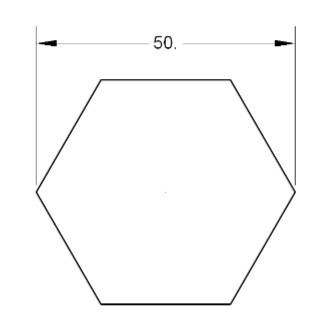As a full turn circle is 360 degrees, we know that the angle between each corner is 60 degrees (360/6).

Let us use the Polar Coordinates Calculator to create a program to mill out the contour.

First, press F2 to open the Inventory Browser and add a new milling workpiece with the dimensions X50 Y50 and Z20.Click on the "Insert at cursor" button.Let us move the zero point to the center of the workpiece to make things easier.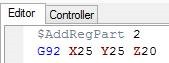As the zero point now is in the center of the workpiece, point number one has the coordinates X-25 Y0.We select a tool and add a G00 block to position there.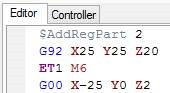Then we go down with G01 to Z-5.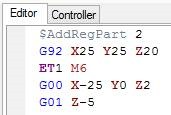Great! Let us calculate the first polar coordinate.

From the Tools menu, under Helpers, select the Polar Coordinates Calculator.As angles are counted from the right side of a circle going around it in a counter-clockwise direction, we get these angles for the hexagon.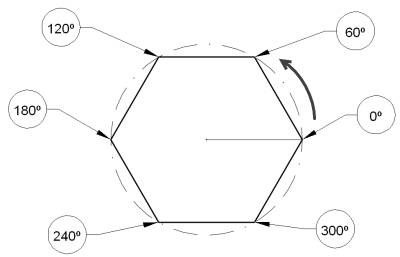In the Polar Coordinates Calculator, we type in X0, Y0 for the reference point as we have moved the zero point to the center. Then we type in 120 for the angle and 25 (the radius) for the distance.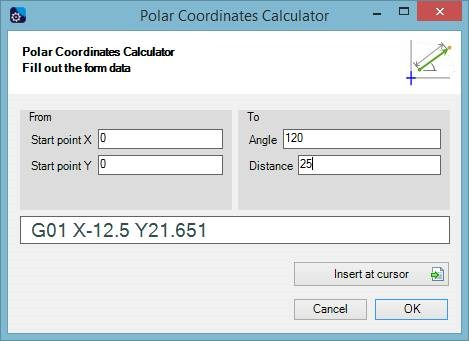As you can see, the calculator has made the G01 block for us. Click the "Insert at cursor" button to send the block to the editor.

You can now simulate the program to check that we get the desired result.Let us go to the next corner at an angle of 60 degrees.

Open the calculator again (shortcut CTRL-O) and note that the current tool position has been filled in for us automatically. In this tutorial, we are not using this feature so put it back to X0 Y0.

This time fill in the angle 60 and the same distance. Once again, click the "Insert at cursor" button to send the calculated block to the editor.

Open the calculator again and set 0 as the angle (X0, Y0, and distance 25 still) and click "Insert at cursor". Repeat with the other angles (300, 240, and back to 180).Congratulations! You have made your first program using the Polar Coordinates Calculator Tool.